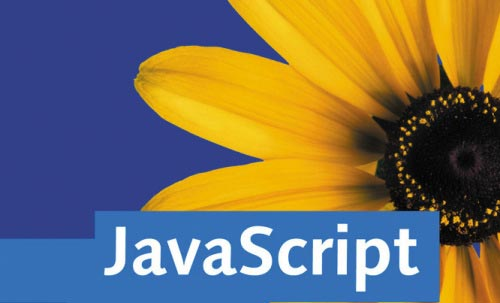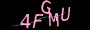×

# 那些年玩转过的一些jQuery开发小技巧### 1. 把你的代码全部放在闭包里面

```(function(\$)
{
//code here
})(jQuery);```

### 2. 提供插件的默认选项

```var defaultSettings = {
mode      : 'Pencil',
lineWidthMin  : '0',
lineWidthMax  : '10',
lineWidth    : '2'
};

settings = \$.extend({}, defaultSettings, settings || {});```

### 3. 使用返回一个元素

JavaScript/jQuery有一个很好的特点就是可以进行方法级联，所以我们不应该破坏这个特性，始终在方法中返回一个元素。我在我的每一个jQuery插件中都遵守这一条。

```\$.fn.wPaint = function(settings)
{
return this.each(function()
{
var elem = \$(this);

//run some code here
}
}```

### 4. 一次性代码放在主循环以外

```var defaultSettings = {
mode      : 'Pencil',
lineWidthMin  : '0',
lineWidthMax  : '10',
lineWidth    : '2'
};

\$.fn.wPaint = function(settings)
{
settings = \$.extend({}, defaultSettings, settings || {});

return this.each(function()
{
var elem = \$(this);

//run some code here
}
}```

### 5. 为什么要设置 Class Prototyping

▲它可以节省很多内存，因为可以不用重复创建这些方法。

▲引用一个现成的方法比重新创建一个好快很多。

### 6. 如何设置 Class Prototyping

```function Canvas(settings)
{
this.settings = settings;
this.draw = false;
this.canvas = null;
this.ctx = null;

return this;
}```

```Canvas.prototype =
{
generate: function()
{
//generate code
}
}```

### 7. 使用 “this” 对象

```Canvas.prototype =
{
generate: function()
{
//some code

var \$this = this;

var buton = //...some code

button.click(function(){
//using this will not be found since it has it's own this

\$this.someFunc(\$this);
});
},

someFunc: function(\$this)
{
//won't know what "this" is.
//use \$this instead passed from the click event
}
}```

### 8. 在每一个对象中保存设置

```function Canvas(settings)
{
this.settings = settings;

return this;
}```

### 9. 分离你的Prototype方法逻辑

```generate()
appendColors()
colorSelect()
colorHoverOn()
colorHoverOff()
appendToElement()
showPalette()
hidePalette()```

### 10. 提供 Setter/Getter 选项

```varlineWidth = \$("#container").wPaint("lineWidth");
\$("#container").wPaint("lineWidth","5");```

```return this.each(function()
{
var elem = \$(this);

var canvas = new Canvas(settings);

//run some code here

elem.data("_wPaint_canvas", canvas);
}
//下面的代码明确了我们究竟要做什么:
\$.fn.wPaint = function(option, settings)
{
if(typeof option === 'object')
{
settings = option;
}
else if(typeof option === 'string')
{
if(
this.data('_wPaint_canvas') &&
defaultSettings[option] !== undefined
){
var canvas = this.data('_wPaint_canvas');

if(settings)
{
canvas.settings[option] = settings;
return true;
}
else
{
return canvas.settings[option];
}
}
else
return false;
}

return this.each(function()
{
//run some code here
}
}```

https://www.jiangweishan.com/article/jQueryNotebook.html

### 网友评论文明上网理性发言已有0人参与